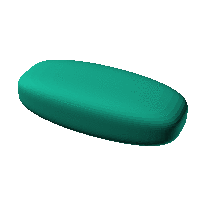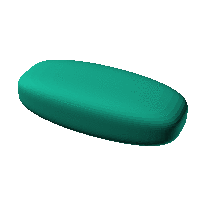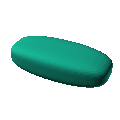Blob Farm# blob58The blob in all its glory:This is an ellipsoid derivative that coudl turn into a rather unimaginative but relatively space efficient case.

 Click on the snapshot to download the blob's stl file.Octave Code:
```  # name of the blob
project = "blob58";

# function at origin must be <0, and >0 far enough away.  w=0 defines the surface
function w = f(x2,y2,z2,c,r)  # three lobed toroid with low profile bumps and flat top
x = (x2-c(1))/r(1);
y = (y2-c(2))/r(2);
z = (z2-c(3))/r(3);

w=((x).^2+(y).^4+(z).^4)-1; # ellipsoid

endfunction;

c_outer = [0,0,0];
r_outer = [180,80,50];

xmin = floor(-200);
xmax = floor(200 );

step = 4;  # grid pitch in mm  start with 4mm to see the shape quickly.  Once you have it just right, change to 2mm for printing

source("../octave/func2stl_v01.m");  # do all the calculations
```
GNU Octave